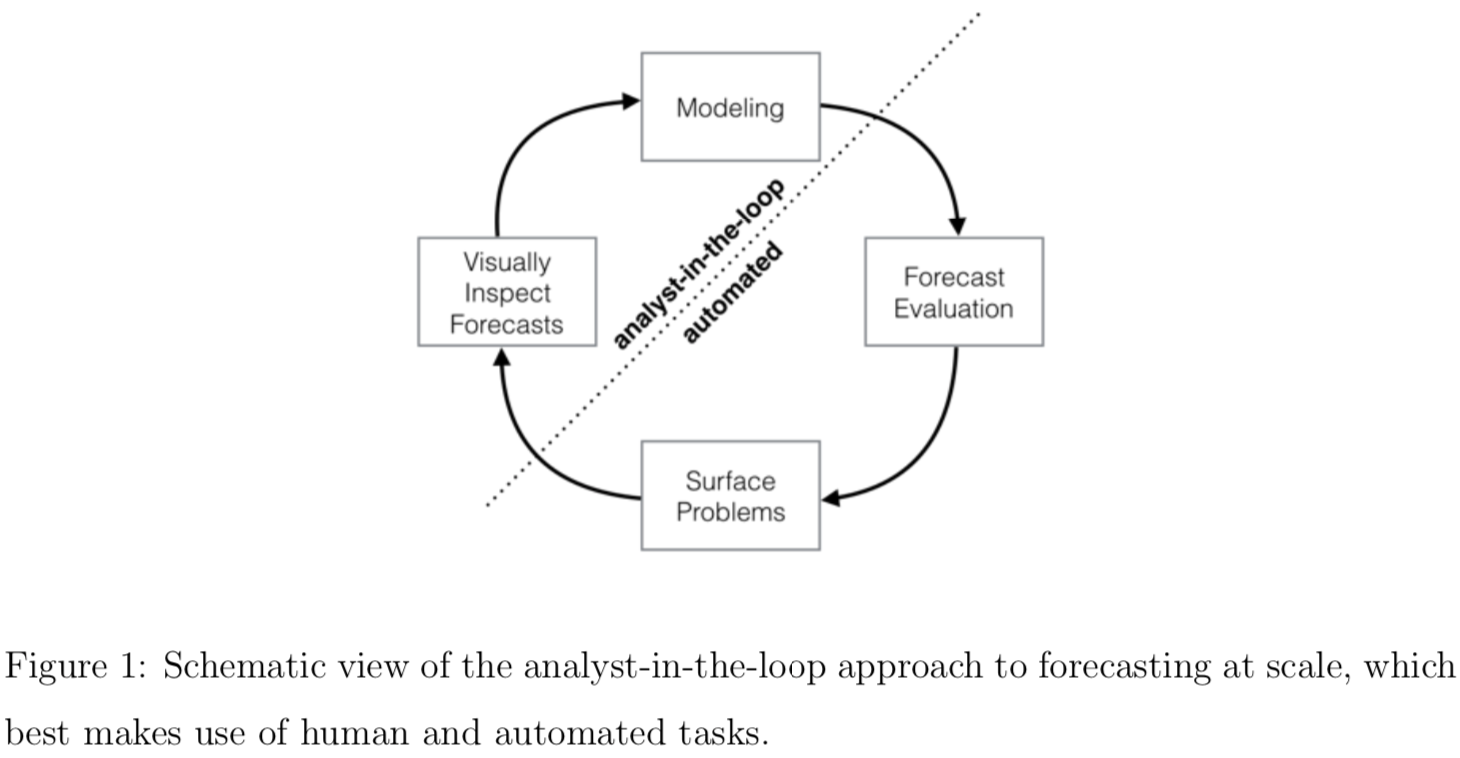# 大规模预测

• 应用
• 商业时序数据预测
• 数据
• 任意时序数据
• 方法
• 时间序列分解模型
• MCMC 建模

## 概要

1. 全自动模型通常难以调参，且过于复杂导致不兼容一些有用的假设；
2. 分析者通常由领域专家担任，他们一般缺少时序数据预测知识方面的训练。

“大规模”的三个含义：

1. 能让没接受过时序数据预测相关训练的人也可以上手；
2. 适应多种多样的预测问题；
3. 自动完成模型评估与对比，分析员只需要通过模型提供的反馈进行调整。

• 周期性影响明显
• 趋势可能改变
• 存在异常点
• 节假日的影响不可忽视### 模型

#### 非周期变化趋势 g(t)

$$g(t) = \frac{C(t)}{1 + \exp{(-k(t) (t - m(t))}}$$

$$g(t) = k(t) t + m(t)$$

#### 周期性变化趋势 s(t)

P 为周期，比较以年为周期时 P=365.25 并建议 N = 10；以星期为周期时 P = 7 并建议 N = 3。

#### 剩余误差项

$$\epsilon_t \sim N(0, \delta)$$